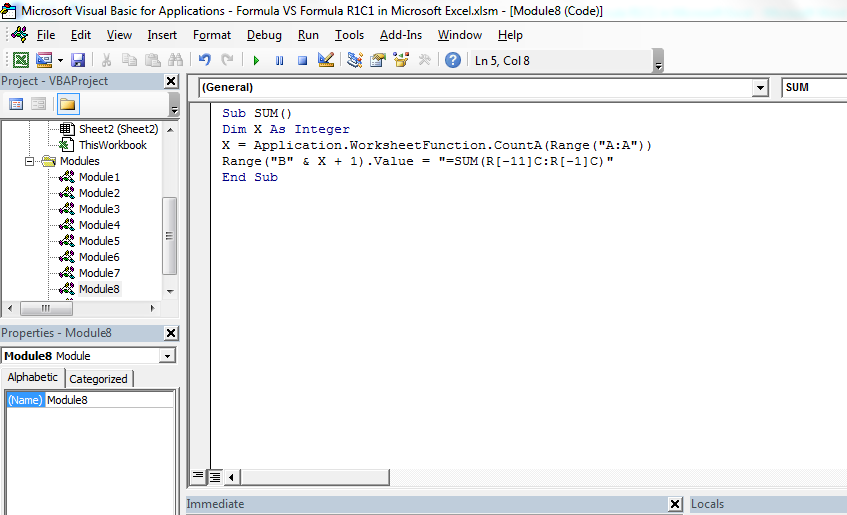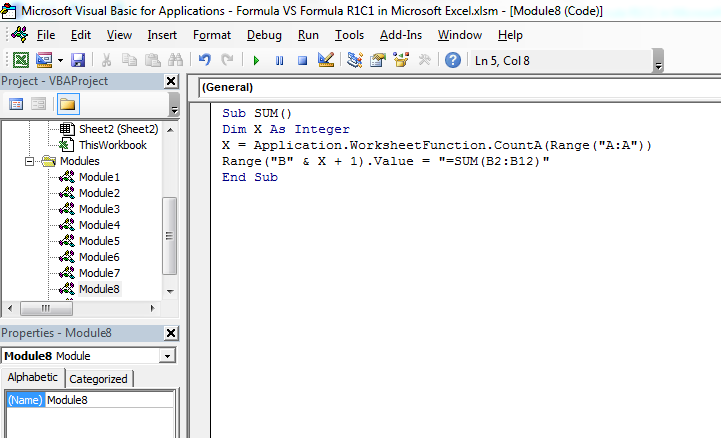Formula VS Formula R1C1 in Microsoft Excel

In this article, we will learn the difference between Formula V/S Formula R1C1 in Microsoft Excel.

In Excel, mostly we use the formula to resolve the problem. But if, for example, we write a UDF that displays the formula of a cell, we might want to display it in the addressing style that is used in the workbook. So then you test to see what addressing style was used and choose between Formula and FormulaR1C1. Possibly, you can also choose between Formula and FormulaLocal (and the R1C1 option of Local).

Formula is best used when you want to set up a relatively straight-forward formula using A1 notation, such as =SUM(A1:A10), or =VLOOKUP(A1,M1:O20,2,False), and you know that the reference cells are locked in position, that is row or column insertion and deletion does not move the formula references. If this happens, it is much harder to set the formula using A1 notation, trying to determine the column letter relative to a known point (such as the cell containing the formula).Same when you use text from the worksheet to construct a formula and write that to a cell.

Let’s take an example:-

• Press the key Alt+F11.
• VBE page will open.
• Write the below mentioned code:-

Sub SUM()

Dim X As Integer

X = Application.WorksheetFunction.CountA(Range(“A:A”))

Range(“B” & X + 1).Value = “=SUM(R[-11]C:R[-1]C)”

End SubYou can write same coding as below mentioned:-

Sub SUM()

Dim X As Integer

X = Application.WorksheetFunction.CountA(Range(“A:A”))

Range(“B” & X + 1).Value = “=SUM(B2:B12)”

End SubCode Explanation: -In the code, we had mentioned that X should be equal to the number of data in a range, so that it remains helpful for defining the range where we need to put the formula.